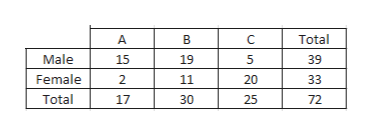# Giving a test to a group of students, the grades and gender are summarized below ABCTotalMale1519539Female2112033Total17302572If one student is chosen at random,Find the probability that the student was female OR got an "B". (give a simplified fraction)

Question
Giving a test to a group of students, the grades and gender are summarized below

 A B C Total Male 15 19 5 39 Female 2 11 20 33 Total 17 30 25 72

If one student is chosen at random,

Find the probability that the student was female OR got an "B". (give a simplified fraction)

check_circleExpert Solution
Step 1

We are given data,help_outlineImage TranscriptioncloseA C Total Male 15 19 5 39 Female 11 20 33 Total 17 30 25 72 fullscreen
Step 2

We have to find,

If one student is chosen, what will be the probability that the student was female OR got an "B"?

There are 33 females total (row3, total column) and 30 people who got a B (look at the bottom of the B column).

So there are 33+30 = 63 people who either are female or got a B.

We need to subtract off the 11 females who got B's. Because it will not take double-counting.

Step 3

So there are 63-11 = 52 people who got a B, they are female, or they are females who got a B grade.

At this point, we are not double coun...

### Want to see the full answer?

See Solution

#### Want to see this answer and more?

Solutions are written by subject experts who are available 24/7. Questions are typically answered within 1 hour*

See Solution
*Response times may vary by subject and question
Tagged in

### Other